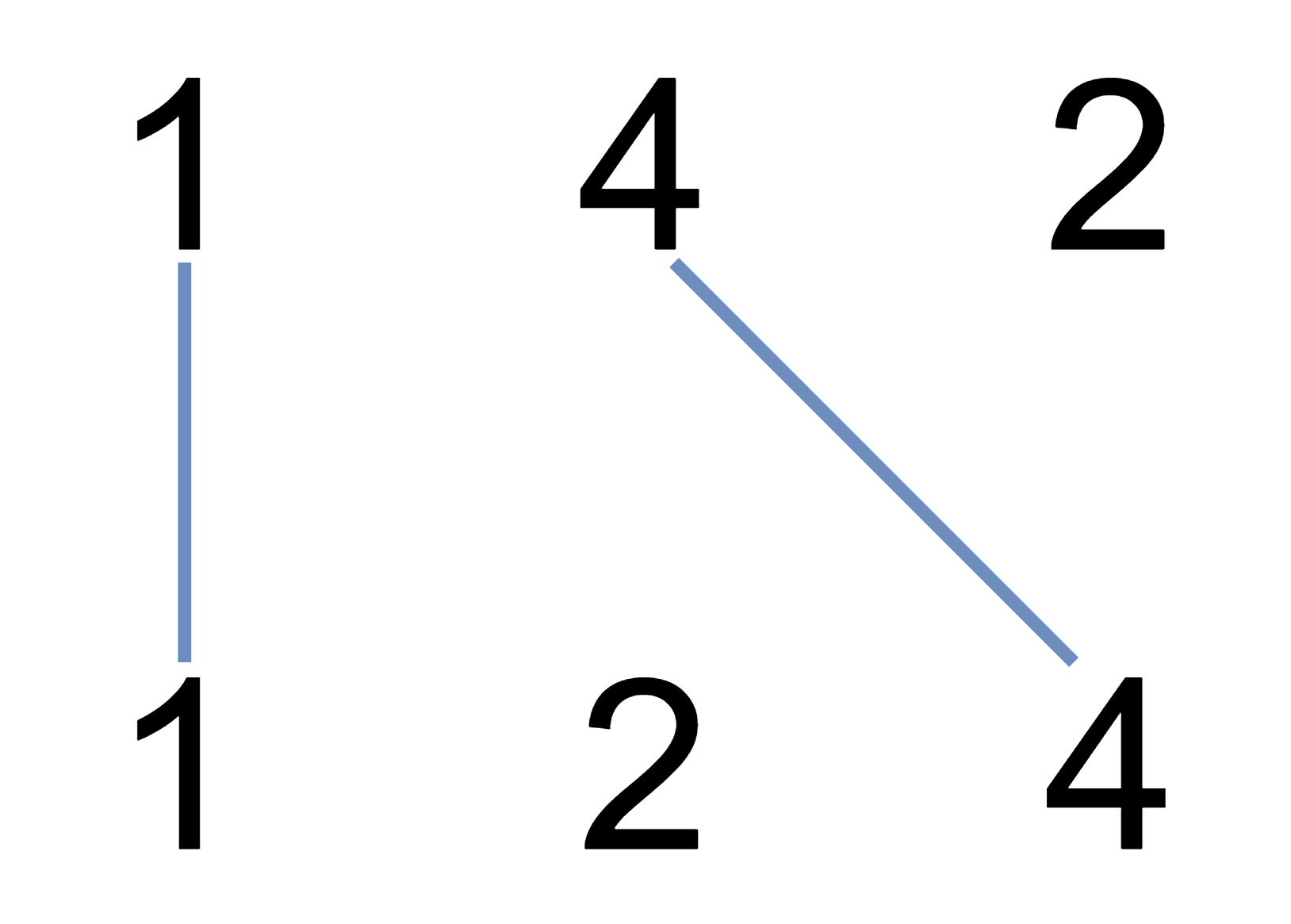We write the integers of `A` and `B` (in the order they are given) on two separate horizontal lines.

Now, we may draw a straight line connecting two numbers `A[i]` and `B[j]` as long as `A[i] == B[j]`, and the line we draw does not intersect any other connecting (non-horizontal) line.

Return the maximum number of connecting lines we can draw in this way.

Example 1:Example 2:

Example 3:

Note:

1. `1 <= A.length <= 500`
2. `1 <= B.length <= 500`
3. `1 <= A[i], B[i] <= 2000`